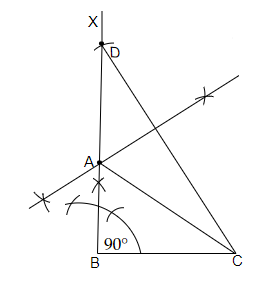# Construct a right triangle whose base is $12 \mathrm{~cm}$ and sum of its hypotenuse and other side is $18 \mathrm{~cm}$.

Given:

Base $=12\ cm$ and the sum of the hypotenuse and another side $=18\ cm$.

To do:

We have to construct the right triangle with the given measurements.

Solution:Steps of construction:

(i) Let us draw the base $BC$ of length $12\ cm$.

(ii) Now, construct an angle $XBC$ such that $\angle XBC=90^o$ from point B.

(iii) Now, by taking the measure $18\ cm$ with the compasses let us draw an arc from point $B$ on the ray $BX$ and mark the point of intersection of the ray with the arc as $D$.

(iv) Let us now join $DC$. Now, draw a perpendicular bisector of the line $CD$ and mark the point of intersection of the bisector with the ray $BX$ as point $A$.

(v) Then join $AC$. Therefore the required right-angled triangle is formed.

Updated on: 10-Oct-2022

23 Views